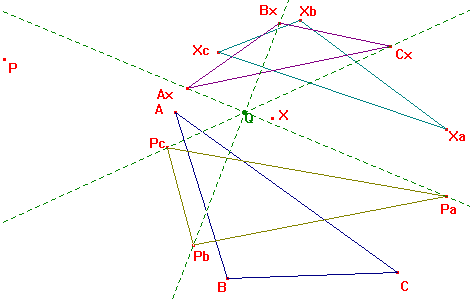r1638

Let X, P be two points; XaXbXc, PaPbPc their circumcevian triangles; AxBxCx the circumcevian triangle of P with respect to XaXbXc. The triangles PaPbPc, AxBxCx are perspective with center Q.
P being the incenter and X being the circumcenter, Q is the point X(56)
P being the circumcenter and X being the incenter, Q is the Bevan point
P being the circumcenter and X being the centroid, Q is the point X(376)
P being the circumcenter and X being the orthocenter, Q is the De Longchamps point
P being the orthocenter and X being the circumcenter, Q is the point X(24)